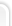## Большая коллекция рефератов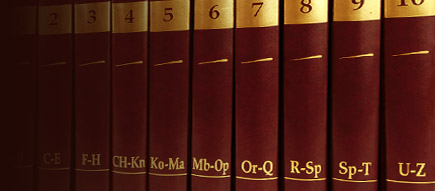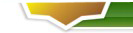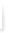### Опросы

Оцените наш сайт?
 Хорошо Отлично Полезно было посетить Добавил в закладки Еще зайду не раз Теперь я здесь постоянный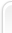# Фрактальные рельефы

{

> Can someone explain the details of how to make game graphics like

> in commanche over kill ?

It's called Voxel. Don't ask me why. Below is a text that explains it.

---------------------------------------------------------------

From: pleung@cs.buffalo.edu (Patrick Leung)

Newsgroups: rec.games.programmer

Subject: How To: Mars Landscape (Repost)

Well, since I posted something about the: 'Voxel landscapes and

How I did it' post by Tim Clarke, a coupla people have asked about

it. So, here it is, Tim Clarke's : Voxel landscapes and How I did it

with some corrections and modifications he added in later postings.

Hope I dont infringe on any of his rights in doing this...

The 'I' s in the following represent Tim Clarke, not me :)

Voxel landscapes and How I did it

---------------------------------

This document describes the method I used in my demo of a Martian terrain,

which can be found at garbo.uwasa.fi:/pc/demo/mars10.zip.

It's similar to a floating horizon hidden line removal algorithm, so you'll

find discussion of the salient points in many computer graphics books. The

difference is the vertical line interpolation.

First, some general points:

---------------------------

The map is a 256x256 grid of points, each having an 8-bit integer height

and a colour. The map wraps round such that, calling w(u,v) the height at

(u,v), then w(0,0)=w(256,0)=w(0,256)=w(256,256). w(1,1)=w(257,257), etc.

Map co-ords: (u,v) co-ordinates that describe a position on the map. The

map can be thought of as a height function h=w(u,v) sampled discretely.

Screen co-ords: (x,y) co-ordinates for a pixel on the screen.

To generate the map:

--------------------

This is a recursive subdivision, or plasma, fractal. You start of with

a random height at (0,0) and therefore also at (256,0), (0,256), (256,256).

Call a routine that takes as input the size and position of a square, in the

first case the entire map.

This routine get the heights from the corners of the square it gets given.

Across each edge (if the map has not been written to at the point halfway

along that edge), it takes the average of the heights of the 2 corners on that

edge, applies some noise proportional to the length of the edge, and writes

the result into the map at a position halfway along the edge. The centre of

the square is the average of the four corners+noise.

The routine then calls itself recursively, splitting each square into four

quadrants, calling itself for each quadrant until the length of the side is

2 pixels.

This is probably old-hat to many people, but the map is made more realistic

by blurring:

w(u,v)=k1*w(u,v)+k2*w(u+3,v-2)+k3*w(u-2,v+4) or something.

Choose k1,k2,k3 such that k1+k2+k3=1. The points at which the map is sampled

for the blurring filter do not really matter - they give different effects,

and you don't need any theoretical reason to choose one lot as long as it

looks good. Of course do everything in fixed point integer arithmetic.

The colours are done so that the sun is on the horizon to the East:

Colour=A*[ w(u+1,v)-w(u,v) ]+B

with A and B chosen so that the full range of the palette is used.

The sky is a similar fractal but without the colour transformation.

How to draw each frame

----------------------

First, draw the sky, and blank off about 50 or so scan lines below the

horizon since the routine may not write to all of them (eg. if you are on top

of a high mountain looking onto a flat plane, the plane will not go to the

horizon).

Now, down to business. The screen is as follows:

---------------------------

| |

| |

| Sky |

| |

| |

|a------------------------| Horizon

| |

| | Point (a)=screen co-ords (0,0)

| Ground | x increases horizontally

| | y increases downwards

| |

---------------------------

Imagine the viewpoint is at a position (p,q,r) where (p,q) are the (u,v)

map co-ordinates and r is the altitude. Now, for each horizontal (constant v)

line of map from v=q+100 (say) down to v=q, do this:

1. Calculate the y co-ordinate of map co-ord (p,v,0) (perspective transform)

you:->------------------------ Horizontal view

:

r :

:

:

-----------------------------P Ground

......................... (q-v)

q v

You have to find where the line between P and you intersects with the

screen (vertical, just in front of 'you'). This is the perspective transform:

y=r/(q-v).

2. Calculate scale factor f which is how many screen pixels high a mountain

of constant height would be if at distance v from q. Therefore, f is small

for map co-ords far away (v>>q) and gets bigger as v comes down towards q.

So, f is a number such that if you multiply a height from the map by f, you

get the number of pixels on the screen high that height would be. For

example, take a spot height of 250 on the map. If this was very close, it

could occupy 500 pixels on the screen (before clipping)->f=2.

3. Work out the map u co-ord corresponding to (0,y). v is constant along

each line.

4. Starting at the calculated (u,v), traverse the screen, incrementing the

x co-ordinate and adding on a constant, c, to u such that (u+c,v) are the map

co-ords corresponding to the screen co-ords (1,y). You then have 256 map

co-ords along a line of constant v. Get the height, w, at each map co-ord and

draw a spot at (x,y-w*f) for all x.

I.e. the further away the scan line is, the more to the "left" u will start,

and the larger c will be (possibly skipping some u columns if c > 1); the

closer the scan line, the lesser u will start on the "left", and c will be

smaller.

Sorry, but that probably doesn't make much sense. Here's an example:

Imagine sometime in the middle of drawing the frame, everything behind a

point (say v=q+50) will have been drawn:

---------------------------

| |

| |

| |

| **** |

| ********* | <- A mountain half-drawn.

|-----**************------|

|*************************|

|********* *********|

|****** ******|

|.........................| <- The row of dots is at screen co-ord y

| | corresponding to an altitude of 0 for that

--------------------------- particular distance v.

Now the screen-scanning routine will get called for v=q+50. It draws in a

point for every x corresponding to heights at map positions (u,v) where u

goes from p-something to p+something, v constant. The routine would put points

at these positions: (ignoring what was there before)

---------------------------

| |

| |

| |

| |

| |

|-------------------------|

| ***** |

| *** *** |

|******* *******|

|.........................|

| |

---------------------------

So, you can see that the screen gets drawn from the back, one vertical

section after another. In fact, there's more to it than drawing one pixel

at every x during the scan - you need to draw a vertical line between

(x,y old) to (x,y new), so you have to have a buffer containing the y values

for every x that were calculated in the previous pass. You interpolate

along this line (Gouraud style) from the old colour to the new colour also,

so you have to keep a buffer of the colours done in the last pass.

Only draw the vertical lines if they are visible (ie. going down,

y new>y old). The screen is drawn from the back so that objects can be drawn

inbetween drawing each vertical section at the appropriate time.

If you need further information or details, mail me or post here... Posting

will allow others to benefit from your points and my replies, though.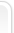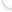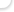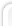Все права защищены © 2010